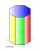# Prism + expression of a variable from the formula - math problems

A prism is a polyhedron comprising an n-sided polygonal base, a second base which is a translated copy (rigidly moved without rotation) of the first, and n other faces (necessarily all parallelograms) joining corresponding sides of the two bases. All cross-sections parallel to the bases are translations of the bases. Prisms are named for their bases, so a prism with a pentagonal base is called a pentagonal prism.

#### Number of problems found: 58

• PrismThe volume of tetrahedral prism is 2.43 m3. Base of prism is a parallelogram in which a side 2,5dm and height ha = 18cm. Calculate the height of the prism.The surface of the regular quadrilateral prism is 8800 cm2, the base edge is 20 cm long. Calculate the volume of the prism
• Prism basesVolume perpendicular quadrilateral prism is 360 cm3. The edges of the base and height of the prism are in the ratio 5:4:2 Determine the area of the base and walls of the prism.The height of a regular quadrilateral prism is v = 10 cm, the deviation of the body diagonal from the base is 60°. Determine the length of the base edges, the surface, and the volume of the prism.
• Triangular prism,The regular triangular prism, whose edges are identical, has a surface of 2514 cm ^ 2 (square). Find the volume of this body in cm3 (l).
• Tetrahedral prismThe height of a regular tetrahedral prism is three times greater than the length of the base edge. Calculate the length of the base edge, if you know that the prism volume is 2187 cm3.
• Base of prismThe base of the perpendicular prism is a rectangular triangle whose legs length are at a 3: 4 ratio. The height of the prism is 2cm smaller than the larger base leg. Determine the volume of the prism if its surface is 468 cm2.
• Regular prismThe regular four-sided prism has a base of 25 cm2 and a surface of 210 cm2. Find its volume.Calculate the volume (V) and the surface (S) of a regular quadrilateral prism whose height is 28.6 cm and the deviation of the body diagonal from the base plane is 50°.
• 3sides prismThe base of vertical prism is an isosceles triangle whose base is 10 cm and the arm is 13 cm long. Prism height is three times the height of base triangle. Calculate the surface area of the prism.
• PrismCalculate the height of the prism having a surface area 448.88 dm² wherein the base is square with a side of 6.2 dm. What will be its volume in hectoliters?
• Pentagonal prismThe regular pentagonal prism is 10 cm high. The radius of the circle of the described base is 8 cm. Calculate the volume and surface area of the prism.
• PrismThe base of a perpendicular triangular prism is a right triangle with legs 4.5 cm and 6 cm long. What is the surface of the prism, if its volume is 54 cubic centimeters?
• Triangular prism - regularThe regular triangular prism is 7 cm high. Its base is an equilateral triangle whose height is 3 cm. Calculate the surface and volume of this prism.The quadrangular prism has a volume of 648 cm3. Trapezoid which is its base has the dimensions bases: a = 10 cm, c = 5 and height v = 6 cm. What is the height of the prism?Calculate the volume and surface area of a regular quadrangular prism 35 cm high and the base diagonal of 22 cm.
• Prism XThe prism with the edges of the lengths x cm, 2x cm, and 3x cm has volume 20250 cm3. What is the area of the surface of the prism?
• Triangular prismThe base of the perpendicular triangular prism is a rectangular triangle with a hypotenuse of 10 cm and one leg of 8 cm. The prism height is 75% of the perimeter of the base. Calculate the volume and surface of the prism.
• Edge of prismThe regular quadrilateral prism has a surface of 250 dm2, its shell has a content of 200 dm2. Calculate its leading edge.
• Octagonal prism vase0.7 l of water can be poured in an octagonal prism vase. What is the height of the vase, if the bottom has a area of 25 cm square and a thickness of 12 mm?

Do you have an interesting mathematical word problem that you can't solve it? Submit a math problem, and we can try to solve it.

We will send a solution to your e-mail address. Solved examples are also published here. Please enter the e-mail correctly and check whether you don't have a full mailbox.

Please do not submit problems from current active competitions such as Mathematical Olympiad, correspondence seminars etc...